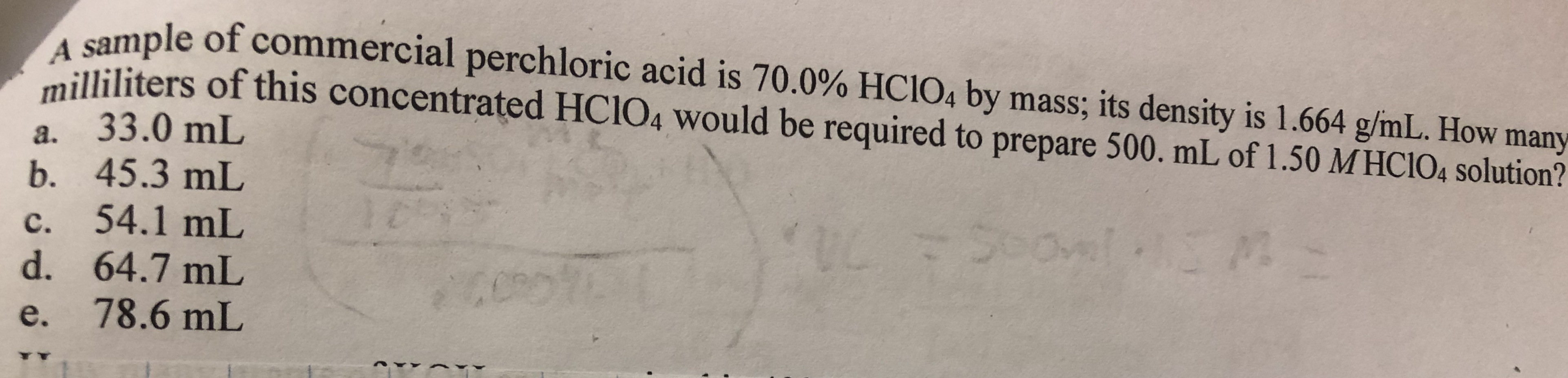le of commercial perchloric acid is 70.0% HCIO4 by mass, its density is 1.664 g/mL. How manyated HClo, would be required to prepare 500 ml of 1.50 МНО 104 solution?illiliters of this concentra. 33.0 mLb. 45.3 mLc. 54.1 mLd. 64.7 mLe. 78.6 mL

Questionhelp_outlineImage Transcriptionclosele of commercial perchloric acid is 70.0% HCIO4 by mass, its density is 1.664 g/mL. How many ated HClo, would be required to prepare 500 ml of 1.50 МНО 104 solution? illiliters of this concentr a. 33.0 mL b. 45.3 mL c. 54.1 mL d. 64.7 mL e. 78.6 mL fullscreen
Step 1

Calculate the mass of perchloric acid in the given solution,

Step 2

Calculate the strength of perchloric acid in the given solution,

Step 3

The normality equatio...

Want to see the full answer?

See Solution

Want to see this answer and more?

Our solutions are written by experts, many with advanced degrees, and available 24/7

See Solution
Tagged in

General Chemistry